# Problem: A heavy flywheel is accelerated (rotationally) by a motor that provides constant torque and therefore a constant angular acceleration . The flywheel is assumed to be at rest at timea) Find the time  it takes to accelerate the flywheel to  if the angular acceleration is .Express your answer in terms of and .b)Find the angle  through which the flywheel will have turned during the time it takes for it to accelerate from rest up to angular velocity.Express your answer in terms of some or all of the following: , , and .Find the angle  through which the flywheel will have turned during the time it takes for it to accelerate from rest up to angular velocity.Express your answer in terms of some or all of the following: , , and .

###### FREE Expert Solution

a)

Constant angular acceleration equation:

ω1 = ω0 + αt

100% (176 ratings)###### Problem Details

A heavy flywheel is accelerated (rotationally) by a motor that provides constant torque and therefore a constant angular acceleration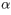. The flywheel is assumed to be at rest at time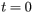a) Find the time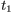it takes to accelerate the flywheel to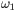if the angular acceleration is.

Express your answer in terms ofand.

b)

Find the angle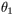through which the flywheel will have turned during the time it takes for it to accelerate from rest up to angular velocity.

Express your answer in terms of some or all of the following:,, and.

Find the anglethrough which the flywheel will have turned during the time it takes for it to accelerate from rest up to angular velocity.

Express your answer in terms of some or all of the following:,, and.